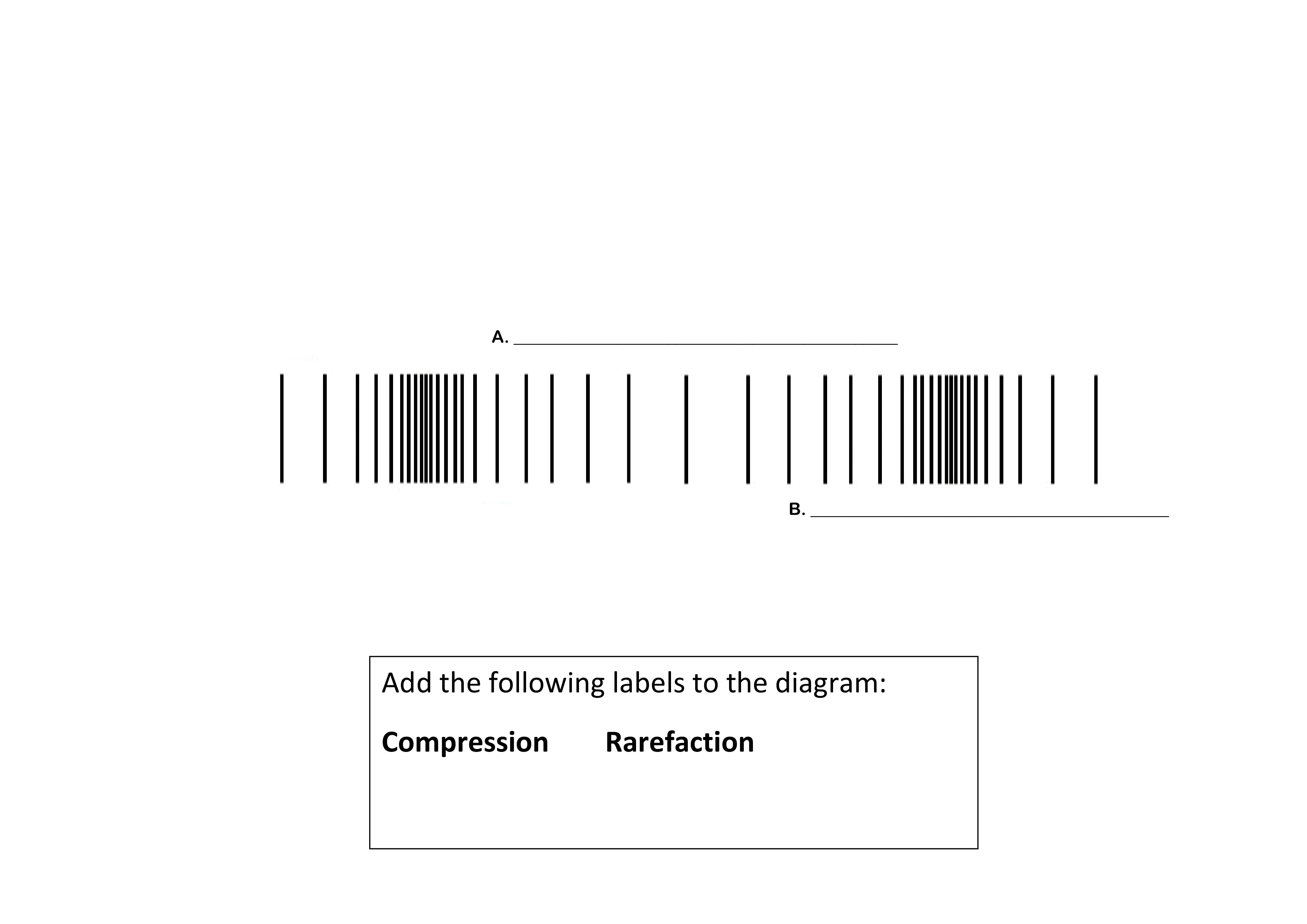6.P.1.1
starstarstarstarstarstarstarstarstarstar
by Mary Hughes
| 6 Questionsa
b
c
d
e
f
Label the parts:

A. ____________________________

B. ____________________________

C. ____________________________

D. ____________________________

E. ____________________________

F. Name this wavea
b
c
Label the parts:

A.. __________________________________

B. __________________________________

C. Name this wave

1
1
A ___________________________ is a disturbance that transfers energy from one place to another.
A. man
B. tranverse
C. wave
D. longitudinal
2
1
Waves can transfer energy through which types of mediums? Write your answer in a complete sentence.
3
1
________________________________ is the number of waves passing a fixed point in a certain amount of time.
A. Frequency
B. Amplitude
C. Resting Point
D. Wavelength
4
1
_______________________ is the distance from one crest or trough to the next.
A. Frequency
B. Amplitude
C. Resting Point
D. Wavelength
5
1
____________________________ is the distance a medium moves above or below its position at rest.
A. Frequency
B. Amplitude
C. Resting Point
D. Wavelength
6
1
The speed of any wave can be determined when both the frequency and the wavelength are known, using the following formular: Speed = wavelength X frequency. Using this information answer the following question: An ocean wave has a wavelength of 16 meters and a frequency of 0.31 wave per second. What is the speed of the wave? Show your work below.